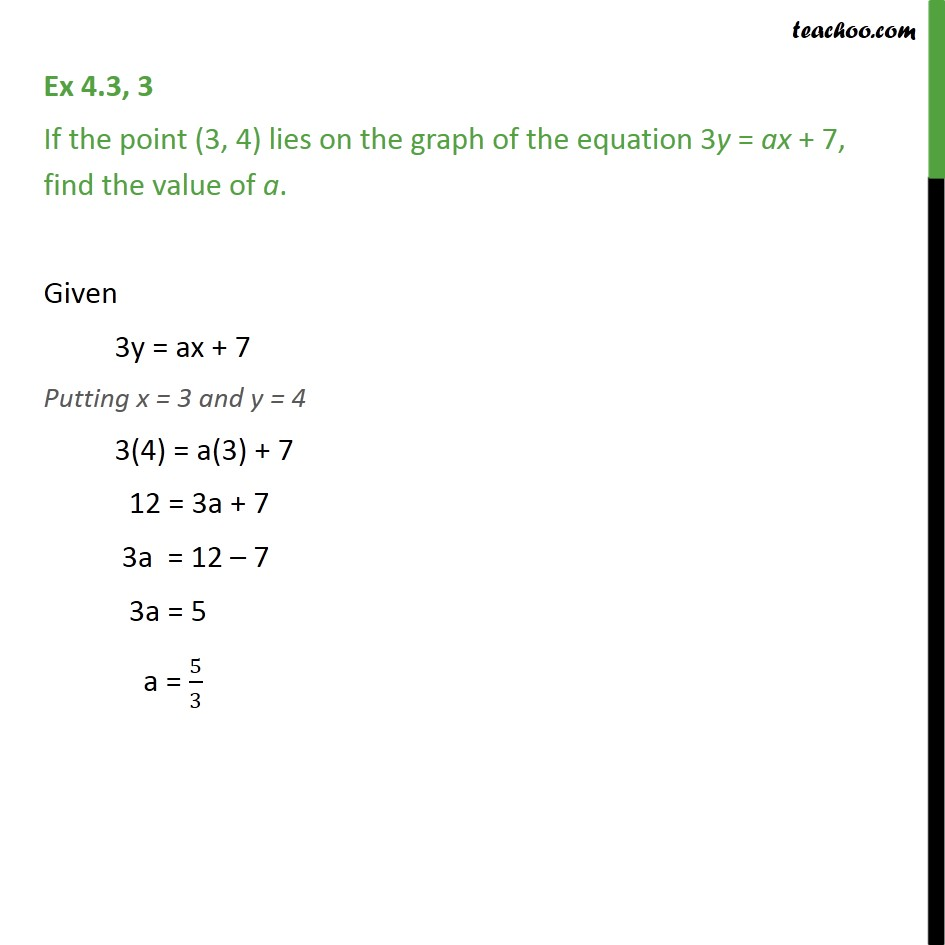Subscribe to our Youtube Channel - https://you.tube/teachoo

1. Chapter 4 Class 9 Linear Equations in Two Variables
2. Concept wise
3. Solution of linear equation

Transcript

Ex 4.3, 3 If the point (3, 4) lies on the graph of the equation 3y = ax + 7, find the value of a. Given 3y = ax + 7 Putting x = 3 and y = 4 3(4) = a(3) + 7 12 = 3a + 7 3a = 12 – 7 3a = 5 a = 5/3

Solution of linear equation